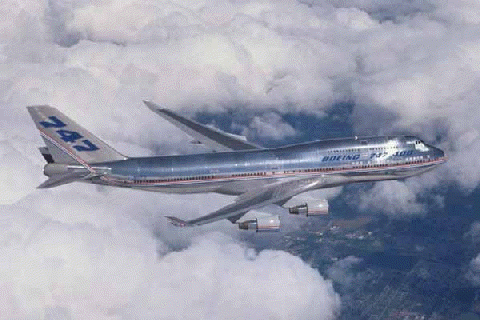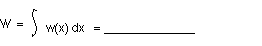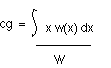+ Text Only Site
+ Non-Flash Version
+ Contact GlennDetermining Center of Gravity (Level 2) Activity If so instructed by your teacher, print out a worksheet page for these problems. Open the slide called Determining Center of Gravity (with text) and read the explanation on how an airplane in flight will rotate about a point in the airplane called the center of gravity. A Boeing 747-400 domestic airplane is soaring the friendly skies (shown below). Use data from the Boeing 747 Wikipedia Website to complete Table 1. Estimate values for data you are unable to locate in a reasonable amount of time. You willl have to select an engine to go on your aircraft. You should be able to find the length of the airplane. Use the BACK key to return to this page. All problems are expressed in metric units.What is the length of a Boeing 747-400 domestic airplane? Length = __________ Let us suppose that the approximate weight distribution (function) on a loaded 747-400 domestic airplane is given by the equation, w(x) = 700 sin(2px / L) + 42,850, where x is the distance measured from the nose of the airplane. Graph the weight distribution. What is the total weight W of the airplane?Use the equation,to calculate the center of gravity of the airplane. The integration is calculated for x = 0 (nose) to Length (tail). [ Hint: Use integration by parts.] cg = __________________ Let us suppose that the approximate weight distribution (function) on a loaded 747-400 domestic airplane is given by the equation, w(x) = 777 ( cos(2px / L) + sin(2px / L) ) + 42,850, where x is the distance measured from the nose of the airplane. Graph the weight distribution. What is the total weight W of the airplane?How does the weight calculated in Problem 3 compare with the weight in Problem 6? Explain why this is so. Use the equation,to calculate the center of gravity of the airplane with the new weight distribution. The integration is calculated for x = 0 (nose) to Length (tail). [ Hint: Use integration by parts.] cg = _____________ Did the center of gravity move? If so, did it move toward the airplane's nose or tail? If not, why did it stay in the same position? If the airplane's center of gravity moved significantly during flight, do you think the pilot would notice it? Why or why not?

Related Pages:
Standards
Worksheet
Lesson Index
Aerodynamics Index+ Inspector General Hotline + Equal Employment Opportunity Data Posted Pursuant to the No Fear Act + Budgets, Strategic Plans and Accountability Reports + Freedom of Information Act + The President's Management Agenda + NASA Privacy Statement, Disclaimer, and Accessibility CertificationEditor: Tom Benson NASA Official: Tom Benson Last Updated: Thu, May 13 02:38:26 PM EDT 2021 + Contact Glenn